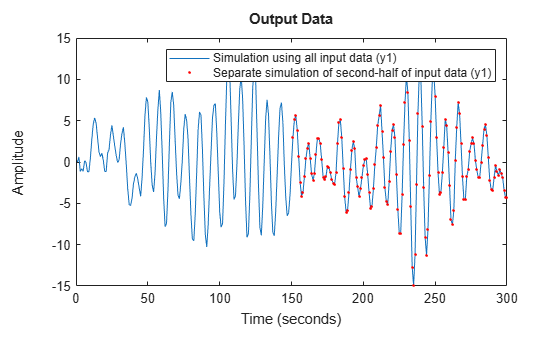data2state

Map past data to states of state-space and nonlinear ARX models

Description

example

X = data2state(sys,PastData) maps the past data to the states of a state-space or a nonlinear ARX model sys. X contains the state values at the time instant immediately after the most recent data sample in PastData. The software computes the state estimates by minimizing the 1-step ahead prediction error between predicted response and output signal in PastData.

data2state is useful for continued model simulation. That is, suppose you have simulated a model up to a certain time instant and would like to then simulate the model for future inputs. Use data2state to estimate states of the model at the beginning of the second simulation.

example

[X,XCov] = data2state(sys,PastData) returns the estimated covariance, XCov, of the current states.

Examples

collapse all

Compute the mapped states of an identified model, and use the states as initial state values for model simulation.

Estimate a second-order state-space model using the data.

sys = ssest(z3,2);

Simulate the model using the entire input signal in z3.

Input = z3(:,[],:); % |iddata| object containing only the input signal
y_all = sim(sys,Input);

sim uses zero initial conditions to compute y_all.

Now simulate the model using only the first-half of the input signal.

Input1 = Input(1:150);
y_1 = sim(sys,Input1);

Continue the simulation with the second-half of the input signal such that the results show no discontinuity owing to initial-condition-induced transients. To do so, first construct a past data set comprising of the input and simulated output from the first-half of the input signal. Then calculate the state values corresponding to the start of the second-half of the input signal (t = 151).

PastData = [y_1,Input1];
X = data2state(sys,PastData);

X contains the state values at the time instant immediately after the most recent data sample in PastData. This time point is also the start of the future data (second-half of the input signal).

FutureData = Input(151:end);

Simulate the model using the second-half of the input signal and X as initial conditions.

opt = simOptions('InitialCondition',X);
y_2 = sim(sys,FutureData,opt);

Verify that y_2 matches the second half of y_all.

plot(y_all,y_2,'r.')
legend('Simulation using all input data',...
'Separate simulation of second-half of input data')PastData = z1;

Estimate an ARX model.

sys = arx(PastData,[1 1 0]);

Convert the model to a state-space model.

sys2 = idss(sys);

Calculate the mapped states and covariance of states using PastData.

[X,XCov] = data2state(sys2,PastData);

X is the state value at the time instant immediately after the most recent data sample in PastData.

z = iddata(y,u,0.02,'Name','Motorized Camera','TimeUnit','s');

Estimate a nonlinear ARX model.

mw1 = nlarx(z,[ones(2,2),ones(2,6),ones(2,6)],'idWaveletNetwork');

The estimated model has six inputs and two outputs.

Determine the model order, nx.

nx = order(mw1);

Use the first nx samples of data to generate initial conditions.

PastData = struct('Input', z.u(1:nx,:),'Output',z.y(1:nx,:));

Compute the mapped states of the model.

X = data2state(mw1,PastData);

X is the state value at the time instant immediately after the most recent data sample in PastData.

Simulate the model using the remaining input data, and specify the initial conditions for simulation.

InputSignal = z.u(nx+1:end,:);
opt = simOptions;
opt.InitialCondition = X;
sim(mw1,InputSignal,opt)Input Arguments

collapse all

Identified model whose current states are estimated, specified as one of the following:

Past input-output data, specified as one of the following:

• iddata object — The number of samples must be greater than or equal to the model order. To determine model order, use order.

X is the value of model states at time PastData.SamplingInstants(end) + PastData.Ts.

When sys is continuous-time, specify PastData as an iddata object. X then corresponds to the discretized (c2d) version of the model, where the discretization method is stored in the InterSampleproperty of PastData.

• Structure — Specified as a structure with the following fields:

• Input — Past input data, specified as an N-by-Nu matrix, where N is great than or equal to the model order.

• Output — Past output data, specified as an N-by-Ny matrix, where N is great than or equal to the model order.

Specify PastData as a structure only when sys is a discrete-time model.

The data samples in PastData should be in the order of increasing time. That is, the last row in PastData should correspond to the latest time.

Output Arguments

collapse all

Mapped states of model, returned as a row vector of size equal to the number of states. X contains the state value at the time instant immediately after the most recent data sample in PastData. That is, if PastData is an iddata object, X is the state value at time t = PastData.SamplingInstants(end)+PastData.Ts.

Estimated covariance of state values, returned as a matrix of size Nx-by-Nx, where Nx is the number of states.

XCov is empty if sys is a nonlinear ARX model.